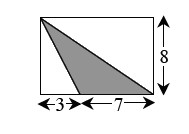### Home > GC > Chapter 2 > Lesson 2.2.2 > Problem2-71

2-71.

The shaded triangle below is surrounded by a rectangle. Find the area of the triangle.The formula to find the area of a triangle is

$\frac{1}{2}(\text{base} \times \text{height})$

$28$ square units.